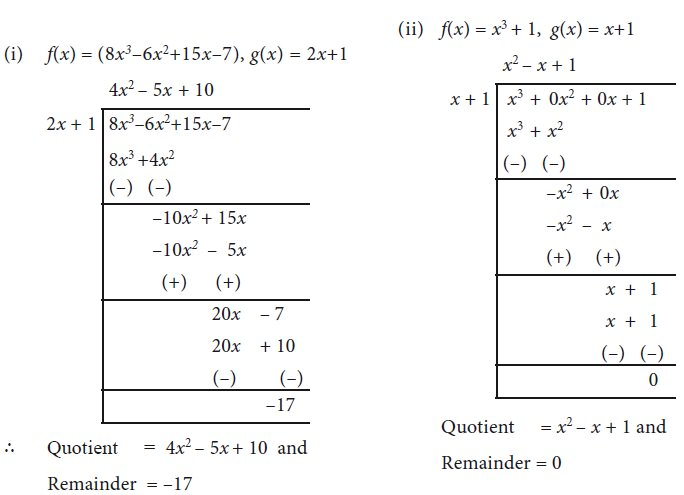Home | Division of Polynomials

# Division of Polynomials

Let us consider the numbers 13 and 5. When 13 is divided by 5 what is the quotient and remainder.?

Division of Polynomials

Let us consider the numbers 13 and 5. When 13 is divided by 5 what is the quotient and remainder.?

Yes, of course, the quotient is 2 and the remainder is3.We write 13 = (5×2)+3

Let us try.From the above examples, we observe that the remainder is less than the divisor.

Can we say ? that when the remainder is 0, then the dividend is the multiple of the divisor?

Dividend = ( Divisor × Quotient ) + Remainder.

Is it possible to divide one polynomial by another ?

Of course, yes, and the way to do it is just the way similar to what you do with numbers!

Let us start with the division of a polynomial by a monomial.

### Example 3.11

Divide x3 -4x2 + 6x by x, where , x 0

Solution

We have## 1. Division Algorithm for Polynomials

Let p(x) and g(x) be two polynomials such that degree of p(x) degree of g(x) and g(x) 0. Then there exists unique polynomials q(x) and r(x) such that

p(x) = g (x) x q(x) + r(x)  … (1)

where r(x) = 0 or degree of r(x) < degree of g(x).

The polynomial p(x) is the Dividend, g(x) is the Divisor, q(x) is the Quotient and r(x)  is the Remainder. Now (1) can be written as

Dividend = ( Divisor × Quotient ) + Remainder.

If r(x) is zero, then we say p(x) is a multiple of g(x). In other  words, g(x) divides p(x).

If it looks complicated, don’t worry ! it is important to know how to divide polynomials, and that comes easily with practice. The examples below will help you.

### Example 3.12

Find the quotient and the remainder when (5x2-7x+2) / (x-1)

Solution### Example 3.13

Find quotient and the remainder when f(x) is divided by g(x)

(i) f(x) = (8x3–6x2+15x–7), g(x) = 2x+1.  (ii) f(x) = x3 + 1, g(x) = x+1

Solution### Example 3.14

If x4 –3x3 + 5x2 –7 is divided by x2 + x + 1 then find the quotient and the remainder.

SolutionTags : Solved Example Problems | Mathematics , 9th EM Mathematics : Algebra
Study Material, Lecturing Notes, Assignment, Reference, Wiki description explanation, brief detail
9th EM Mathematics : Algebra : Division of Polynomials | Solved Example Problems | Mathematics